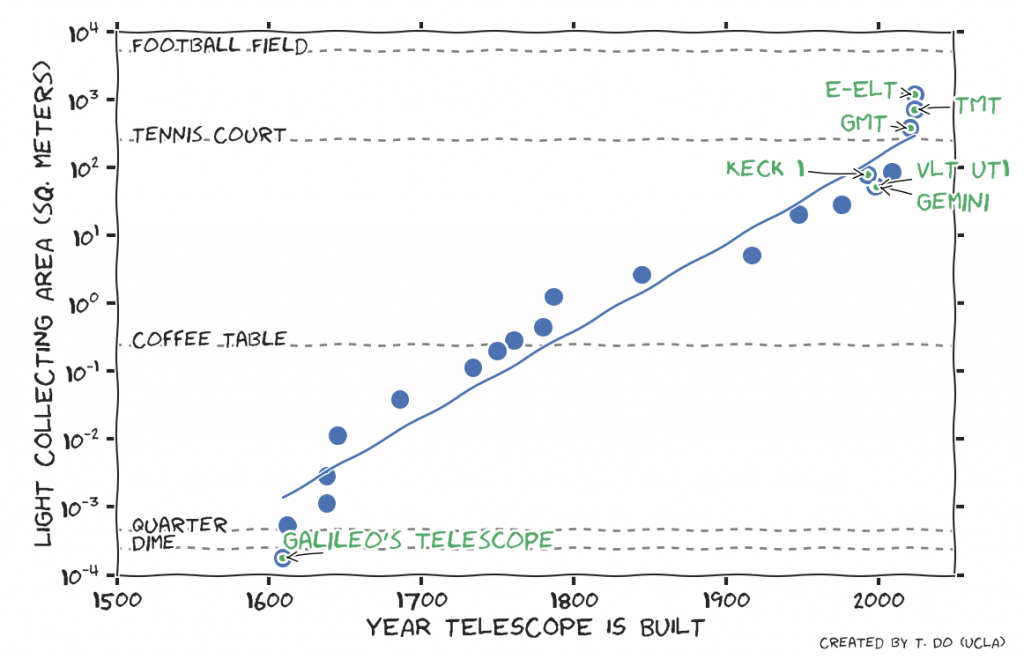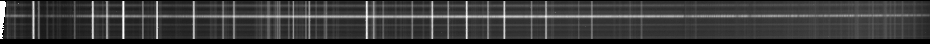## How to find indices of the intersection of two numpy arrays

Given numpy arrays a and b, it is fairly straightforward find the indices of array a whose elements overlap with the elements of array b using the function numpy.in1d(). However, what if you want to also find the indices associated with elements in b that overlap with a? I’m unable to find a good solution to this, so I wrote a small function to do this. It does brute force matching after getting all the common elements of array a and b. If anyone knows of a better way, please let me know. The code snippet is below.

```import numpy as np

def overlap(a, b):
# return the indices in a that overlap with b, also returns
# the corresponding index in b only works if both a and b are unique!
# This is not very efficient but it works
bool_a = np.in1d(a,b)
ind_a = np.arange(len(a))
ind_a = ind_a[bool_a]

ind_b = np.array([np.argwhere(b == a[x]) for x in ind_a]).flatten()
return ind_a,ind_b
```

Usage:

```import overlap

a = np.array([1,2,4,5])
b = np.array([4,6,10,9,1])

ind_a, ind_b = overlap.overlap(a,b)
```

UPDATE (2016-09-28): Based on comments from Mike (see below), there is different way to do this to account for non-unique elements. This also likely runs faster too. His suggestion is below:

```def overlap_mbk(a, b):
a1=np.argsort(a)
b1=np.argsort(b)
# use searchsorted:
sort_left_a=a[a1].searchsorted(b[b1], side='left')
sort_right_a=a[a1].searchsorted(b[b1], side='right')
#
sort_left_b=b[b1].searchsorted(a[a1], side='left')
sort_right_b=b[b1].searchsorted(a[a1], side='right')

# # which values are in b but not in a?
# inds_b=(sort_right_a-sort_left_a == 0).nonzero()
# # which values are in b but not in a?
# inds_a=(sort_right_b-sort_left_b == 0).nonzero()

# which values of b are also in a?
inds_b=(sort_right_a-sort_left_a > 0).nonzero()
# which values of a are also in b?
inds_a=(sort_right_b-sort_left_b > 0).nonzero()

return a1[inds_a], b1[inds_b]
```

## Moore’s Law for Telescopes: Light collecting area of telescopes doubles every 20 years

by T. DoOne of the most important changes in telescopes over time is their size. Larger telescopes collect more light, which lets us see fainter and farther objects. Galileo’s first telescope had a 1.5 cm diameter opening to let light through its lenses. This is slightly smaller than a dime. Using this telescope he discovered the moons of Jupiter. Today, the largest optical and near-infrared telescopes are imaging Jupiter-sized planets in other solar systems, and seeing light from some of the first galaxies. The next generation of telescopes coming in about 10 years, like TMT, E-ELT, and GMT are predicted to see the first stars in the universe.

This is possible through the tremendous pace of technological progress. The above plot uses data tabulated on Wikipedia to show the light collecting area of large telescopes at the time they were built. Between Galileo’s telescope and the Keck telescopes, their diameters have grown by over a factor of 600, while their area has grown by over 400,000 times. The line in the plot is a exponential fit to the relationship between time a telescope was complete and the collecting area (Area = Ao exp(time/Tau)). This means that the area of telescopes have doubled roughly about once every 20 years over the 400 year history of telescopes. This trend is not always smooth as there can be a large jump in the size of the mirrors between generations of telescopes. For example, the jump from Keck (1993) to TMT (2024) will represents a change from 10 m to 30 m, which corresponds to a factor of 9 increase in area in 31 years.

## Near-infrared stellar absorption line list from Arcturus

T. Do

The star Arcturus is among the brightest infrared sources in the sky, which makes it possible to observe the star at very high spectral resolutions. The infrared spectrum of Arcturus serves as a standard for our understanding of red giants (Arcturus is a K0III star). A very comprehensive spectral atlas of Arcturus was published by Hinkle, Walllace, and Livingston (1995). The observed spectrum is available through VizieR. Here for convenience, I’ve converted the appendices of atomic and molecular lines into comma-separated text files and converted the line centroids into microns. Lines are at vacuum wavelengths.

For convenience, I’ve also included the atomic line list in an html table at the end of the post. It is a very long table, so it will be below the fold. If you have trouble viewing, it may be your javascript settings.

## Testing the MOSFIRE wavelength solution: comparison of arc lamp wavelength solution to sky lines

Tuan Do

In order to determine the accuracy of the MOSFIRE wavelength solution, I compared the solution determined using Ne and Ar lamps with that of the expected positions of OH lines.  This experiment tests a specific night and configuration, so is not meant to be comprehensive.

The dataset comes from observations of the Galactic center on 2012-05-29 and were reduced with an unofficial development version of the MOSFIRE pipeline that was modified to use the arcs to compute the wavelength solution.One of the reduced, rectified slits without sky subtraction. The rectification is based on using the Ne and Ar lamp solution.

## A brief statistical analysis of the Canadian penny phase-out: Are stores now charging more because of rounding?

On February 4th, 2013, the Royal Canada Mint stopped making pennies. This means that effectively soon, cash transactions will have to be rounded to the nearest 5 cents. Example: \$1.01 will be \$1.00, \$1.03 will be \$1.05, and \$1.08 will be \$1.10 (official Canadian guide to this: http://www.fin.gc.ca/1cent/index-eng.asp).  In principle, if the price of an item is random, then on average, all transactions should even out: sometimes the rounding will be to your advantage, sometimes it will be to the store’s. However, prices are not random, as walking through a supermarket will tell you. Very often, prices end in 99 cents. In the worse case scenario then, if you ever only make one transaction at a time, and buy items that end in 99 cents, you will be charged a penny extra each time (if you buy some food item that is not taxed). Generally, this would not be much of a problem, unless there is a systematic statistical bias overall. That would mean that while the rounding procedure is meant to be a “fair and transparent matter”, people would end up paying more inadvertently.

## Python script to extract pages from a postscript file

This script will examine a postscript file and output individual pages as separate ps files. This is useful for separating pages for figures.

``` import subprocess import sys import numpy as np import os```

```if (len(sys.argv) > 1): fileName = sys.argv print fileName callStr = "-c %%Page: "+fileName #print 'calling: '+callStr```

``` process = subprocess.Popen(['grep '+callStr],shell=True,stdout=subprocess.PIPE) pages = process.communicate() pages = int(pages)```

``` print '%i pages to extract' % (pages) for i in np.arange(1,pages+1): tempSplit = os.path.splitext(fileName) tempName = tempSplit+'_page'+str(i)+tempSplit print 'Outputing file: '+tempName subprocess.call(['psselect','-p'+str(i), fileName, tempName])```

## Non-printable characters and ASCII with IDL

Sometimes it is necessary to use non-printable characters in a string or special characters such as the ‘ and ” that normally denote a string in IDL. It is easy to do so by referring to the correct byte presentation using the `string()` function.

For example to put in a tab: `string(9B)`

The apostrophe ‘: `string(39B)`

The quotation mark “: `string(34B)`

The other ASCII characters can be found here: http://en.wikipedia.org/wiki/ASCII – use the Dec. column

## Tcl/tk error in python

In python, if the tcl package is not compiled with the threads option, if it runs a tcl/tk application, it will crash with the following error at the end:
`TclError: out of stack space (infinite loop?)`

To reproduce this, just run the `pydoc.gui()` program:
``` import pydoc pydoc.gui() ```

This problem will affect pyraf and prevent it from loading. To fix this, compile the tcl package with `--enable-threads` or in macports, install the `thread` variant: `port install tcl +threads`.

## Path of a module imported in python

Each module in python has a `.__path__` attribute that shows its path. This is useful if you need to look up the location of a particular module that is loaded in python.